Data Analysis with R

4 - Operators

Postdoctoral Researcher

Operators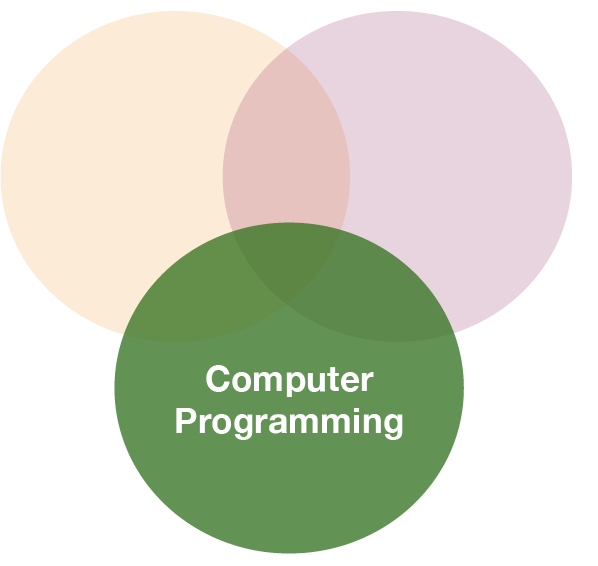Overview of operators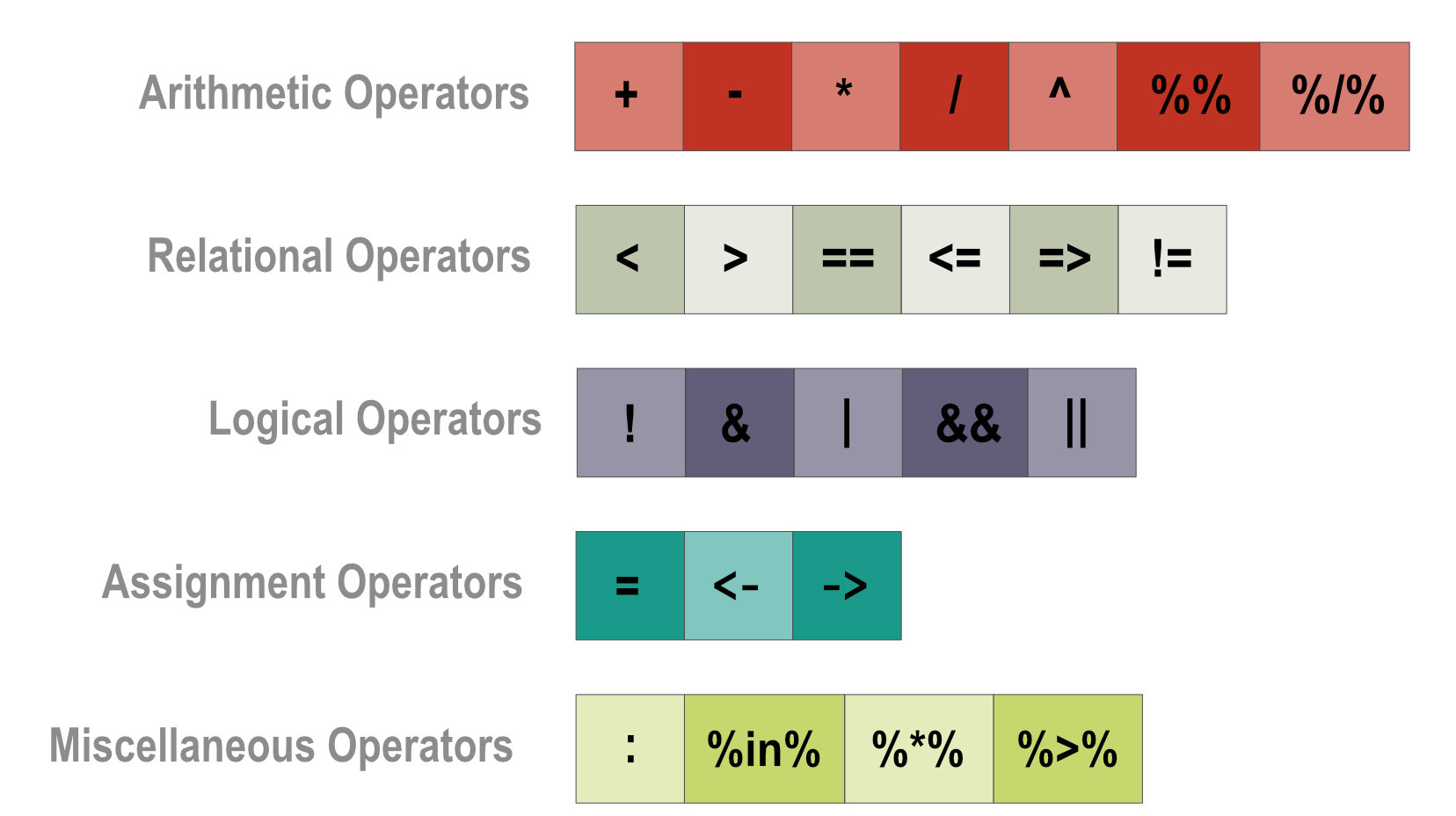Relational operators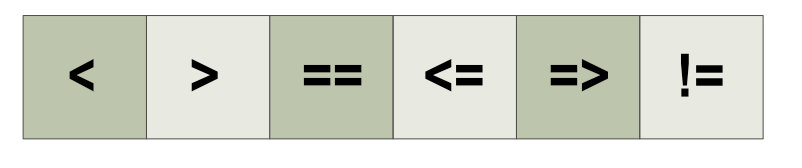• find out relation between two operands
• six relational operations are supported in R
• output is logical (TRUE or FALSE) for all of these operators
• work element-wise
Operator Usage Description
< a < b a is LESS than b
> a > b a is GREATER than b
== a == b a is EQUAL to b
<= a <= b a is LESS than or EQUAL to b
>= a > = b a is GREATER than or EQUAL to b
!= a!=b a is NOT EQUAL to b

Example of relational operators# Example for numbers
a <- 10
b <- 5
print(a < b) # less
print(a >= b) # greater or equal
print(a != b) # not equal

Example of relational operators# Example for numbers
a <- 10
b <- 5
print(a < b) # less
##  FALSE
print(a >= b) # greater or equal
##  TRUE
print(a != b) # not equal
##  TRUE

Example of relational operators# Example for numbers
a <- 10
b <- 5
print(a < b) # less
##  FALSE
print(a >= b) # greater or equal
##  TRUE
print(a != b) # not equal
##  TRUE
# Example for vectors
a <- c(7.5, 3, 5)
b <- c(2, 7, 5)
print ( a <= b ) # less or equal
print ( a != b ) # not equal

Example of relational operators# Example for numbers
a <- 10
b <- 5
print(a < b) # less
##  FALSE
print(a >= b) # greater or equal
##  TRUE
print(a != b) # not equal
##  TRUE
# Example for vectors
a <- c(7.5, 3, 5)
b <- c(2, 7, 5)
print ( a <= b ) # less or equal
##  FALSE  TRUE  TRUE
print ( a != b ) # not equal
##   TRUE  TRUE FALSE

Logical (boolean) operators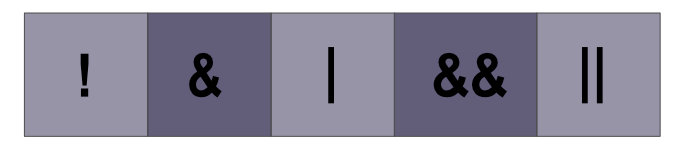• work only for the basic data types (e.g. logical, numeric) and atomic vectors in R.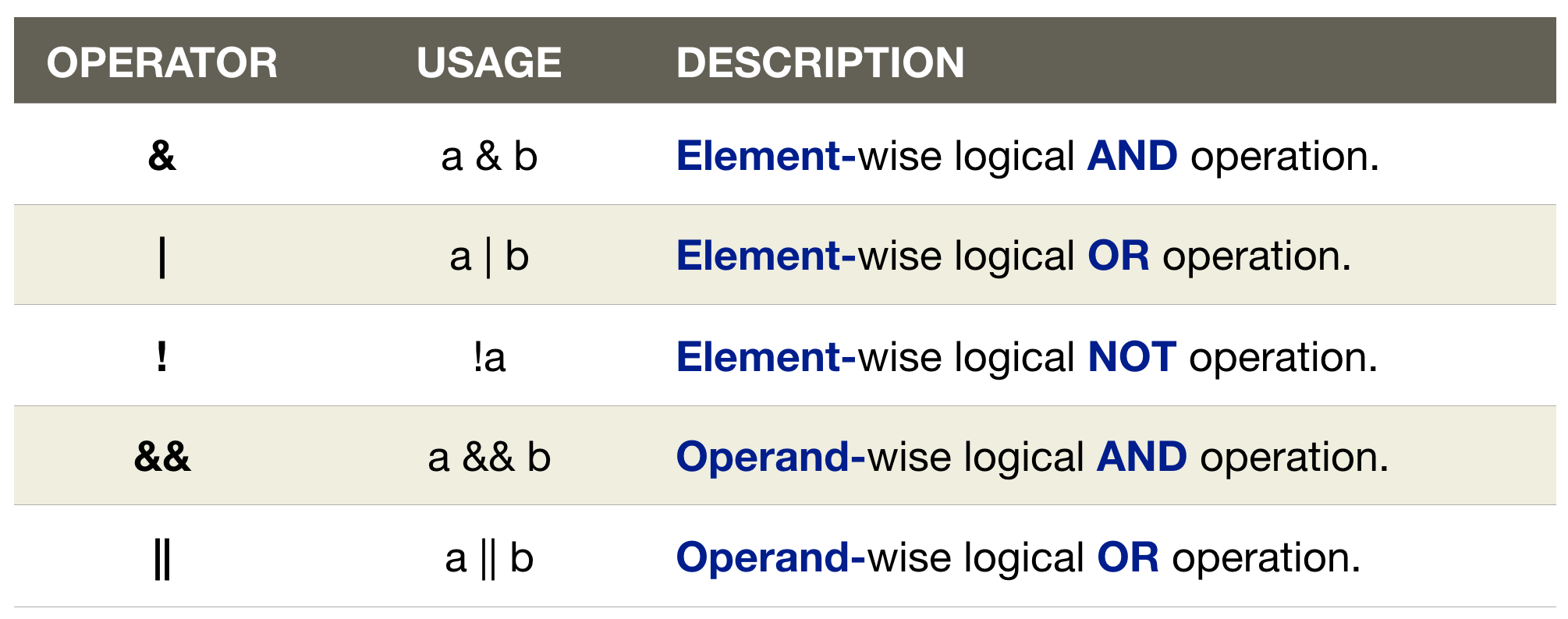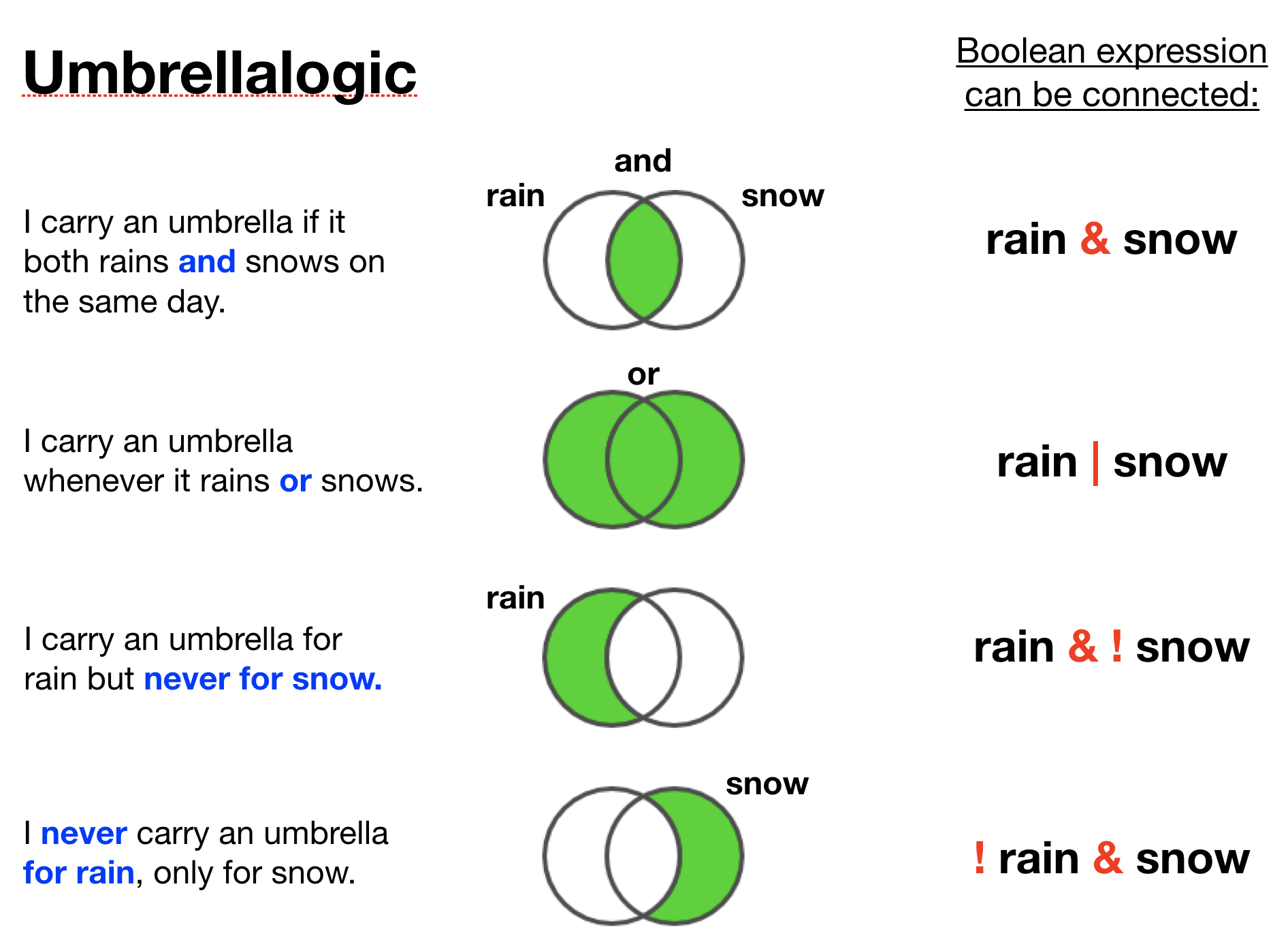Example of combined boolean operatorsx <- 1:5
x[ x < 4 & x >= 2]
##  2 3

Example of combined boolean operatorsx <- 1:5
x[ x < 4 & x >= 2]
##  2 3
Step Usage 1 2 3 4 5
1 x < 4 TRUE TRUE TRUE FALSE FALSE
2
3

Example of combined boolean operatorsx <- 1:5
x[ x < 4 & x >= 2]
##  2 3
Step Usage 1 2 3 4 5
1 x < 4 TRUE TRUE TRUE FALSE FALSE
2 x >= 2 FALSE TRUE TRUE TRUE TRUE
3

Example of combined boolean operatorsx <- 1:5
x[ x < 4 & x >= 2]
##  2 3
Step Usage 1 2 3 4 5
1 x < 4 TRUE TRUE TRUE FALSE FALSE
2 x >= 2 FALSE TRUE TRUE TRUE TRUE
3 x < 4 & x >= 2 FALSE TRUE TRUE FALSE FALSE

Element- vs. operand-wise operationa <- c(TRUE, TRUE, FALSE, FALSE)
b <- c(TRUE, FALSE, TRUE, FALSE)

print(a | b)
##   TRUE  TRUE  TRUE FALSE
print(a || b)
##  TRUE

Other miscellaneous operators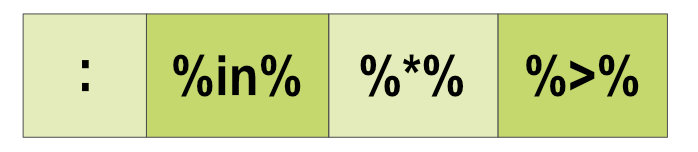• are similarly important for manipulating data.
Operator Usage Description
: a:b Creates series of numbers from left operand to right operand
%in% a %in% b Identifies if an element(a) belongs to a vector(b)
%*% A %*% t(A) Performs multiplication of a vector with its transpose

Other miscellaneous operators• are similarly important for manipulating data.
Operator Usage Description
: a:b Creates series of numbers from left operand to right operand
%in% a %in% b Identifies if an element(a) belongs to a vector(b)
%*% A %*% t(A) Performs multiplication of a vector with its transpose

Example for %in%a <- c(25, 27, 76)
b <- 27
print(b %in% a)
##  TRUE
print(a %in% b)
##  FALSE  TRUE FALSE

Quiz 1: Relational operators

What does the following operation return (try to find the answer without using R):

a <- c(6, 80, 107, 164, 208, 53, 216, 268, 65, 283)
a < 60
1. NA
2. a numerical vector containing 6 and 53
3. TRUE
4. FALSE
5. a logical vector with TRUEs and FALSEs

R checks for each element in a whether its value is less than 60 and returns a TRUE or otherwise a FALSE. As we have 10 elements in a the returned logical vector has also 10 elements.

Quiz 2: Relational operators

How many TRUEs would you get from the following operation (try to find the answer without using R):

a <- c(6, 80, 107, 164, 208, 53, 216, 268, 65, 283)
a <= 80
1. 1
2. 6
3. 4
4. 3

R applies the operation element-wise: 6 <= 80? 80 <= 80? 107 <= 80?...

Four elements have values that are TRUEly less than or equal to 80, i.e. 6,53,65, and 80.

Quiz 3: Relational operators

How many TRUEs would you get from the following operation (try to to find the answer without using R):

a <- c(16, 47, 207)
b <- c(0, 49, 410)
a <= b
1. 1
2. 2
3. 5

R applies the operation element-wise in both vectors: 16 <= 0? 47 <= 49? 207 <= 410?

Two values in a are TRUEly less than or equal to the corresponding values in b, i.e. 16 and 47.

Quiz 4: Relational operators

What do the following operations on these vectors return:

a <- c(4, 5, 1, 8, 8, 10)
b <- c(0, 0, 3, 6, 7, 9); c <- 3
1. a[a < b]
2. b[b == c]
3. sum(c >= b)

If a vector is shorter than the other it gets recycled for the element-wise comparison

1. Recall, a < b returns a logical vector of length a and b (=c(FALSE,FALSE,TRUE,FALSE,FALSE,FALSE)), which you then use to subset the vector a. Only the 3rd element in a (=1) is TRUEly less than the corresponding element in b (=3) and its number is then returned: 1

2. c gets first recycled (meaning that the value 3 gets repeated 6 times) for the element-wise comparison, in which only one element has the same value as c and the value of that element is then returned: 3.

3. c >= b returns a vector with 3 TRUEs and 3 FALSEs. Why? When you calculate the sum, R coerces the elements to integers (recall, TRUE turns into 1 and FALSE into 0), so the correct answer is 3.

Logical operators

For 6 days it was measured whether it was sunny (sunny = TRUE) and whether it was hot (hot = TRUE). Now we want to check for several conditions (try to to find the answer without using R):

sunny <- c(TRUE, TRUE, TRUE, FALSE, FALSE, FALSE)
hot <- c(FALSE, TRUE, FALSE, TRUE, FALSE, TRUE)

Quiz 5: Logical operators

What does the following return?

sunny <- c(TRUE, TRUE, TRUE, FALSE, FALSE, FALSE)
hot <- c(FALSE, TRUE, FALSE, TRUE, FALSE, TRUE)
sunny & hot
1. a vector of length 12 (with 6 TRUEs and 6 FALSEs)
2. a vector of length 6 (with 1 TRUE and 5 FALSEs)
3. a vector of length 6 (with 3 TRUEs and 3 FALSEs)

& is an element-wise AND operator: a TRUE is only returned if it is sunny and hot (both TRUE)

Both vectors have a length of 6 (6 days), hence,the returned vector has also 6 element. It contains only 1 TRUE (in position 2) as only at day 2 the weather was sunny AND hot.

Quiz 6: Logical operators

What does the following return?

sunny <- c(TRUE, TRUE, TRUE, FALSE, FALSE, FALSE)
hot <- c(FALSE, TRUE, FALSE, TRUE, FALSE, TRUE)
sunny | hot
1. a vector with 6 TRUEs
2. a vector with 5 TRUEs and 1 FALSE
3. a vector with 1 TRUE and 5 FALSEs

| is an element-wise OR operator: a TRUE is returned if it is sunny or hot (at least one of both is TRUE).

Every day it was sunny or hot, except for day 5 (hence, here a FALSE in the returned vector).

Quiz 7: Logical operators

What does the following return?

sunny <- c(TRUE, TRUE, TRUE, FALSE, FALSE, FALSE)
hot <- c(FALSE, TRUE, FALSE, TRUE, FALSE, TRUE)
sunny || hot
1. FALSE
2. TRUE

The question to this operation would be: Was is for any of the 6 days at least sunny or hot?

|| carries out a logical OR operation consolidated for all elements: first the OR operation is carried out element-wise and then it is checked whether at least one of the returned element is a TRUE.

Quiz 8: Combining operators

Which values do you get from the following vector:

a <- c(6, 80, 107, 164, 208, 53, 216, 268, 65, 283)
1. a[a > 50 & a < 60]
2. a[a > a & a < a]
3. sum(a > 250 | a < 100)
4. sum(a[a %in% 1:60])
1. 53.
2. 216.
3. 6.
4. 59.

Quiz 9 - Challenge: Using operators for subsetting

df <- data.frame(
sample = letters[1:10],
group = c(rep(1, 5), rep(2, 5)),
value = c(6, 80, 107, 164, 208, 53, 216, 268, 65, 283)
)

Subset this data frame using the operators you just learned:

1. Extract all observations from group 2
2. Extract all observations where values are greater than 150.
3. Extract all observations from group 1 where values are less than 50 or greater than 250.
4. Extract all observations that have the letters "a", "c", "g", or "j"

(for a hint press p and for a solution code see last slide)

p

Well Done!!!! You mastered the most important R basics that are fundamental for all that follows.

You are now able to ...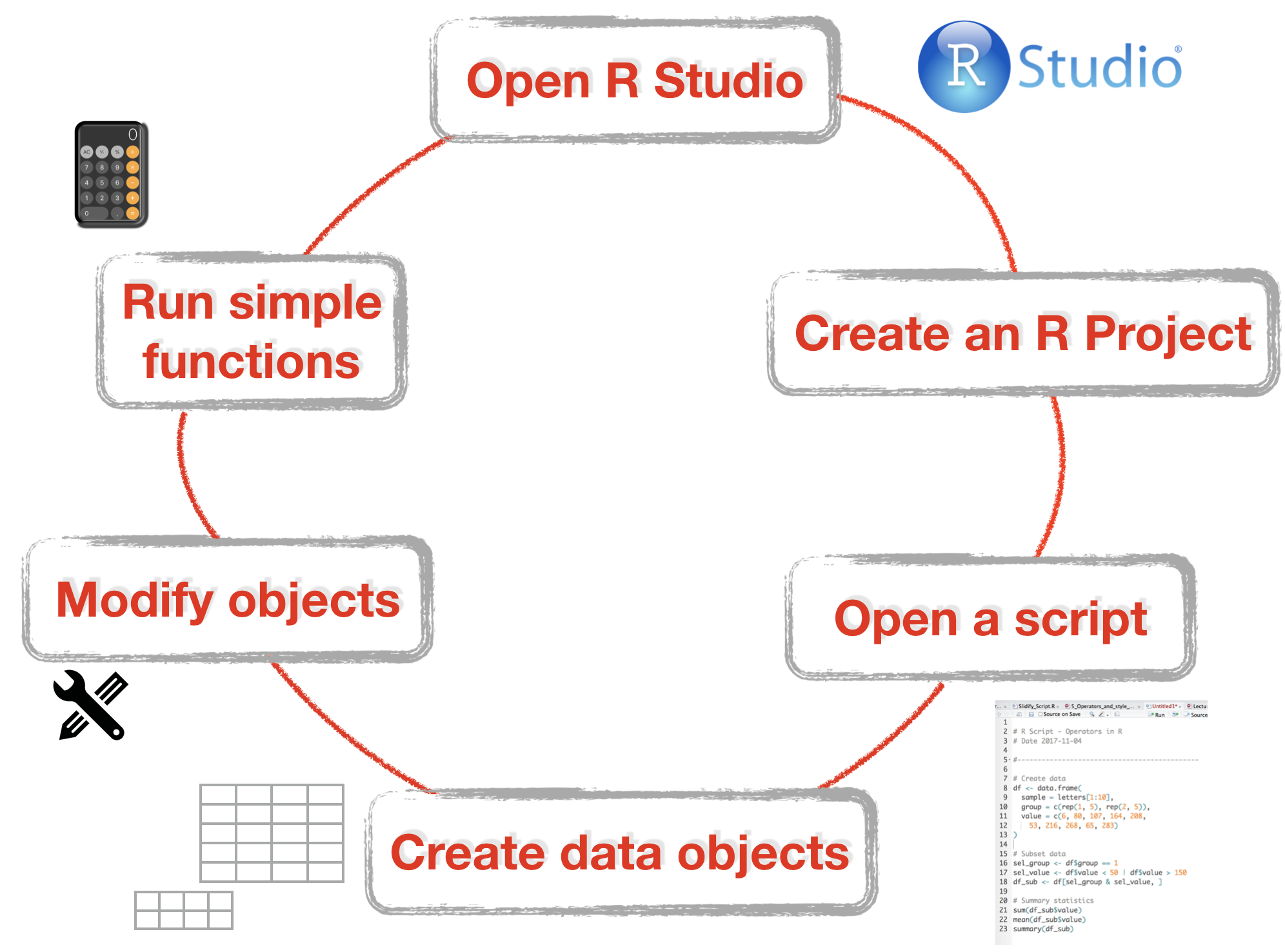How do you feel now.....?

Totally bored?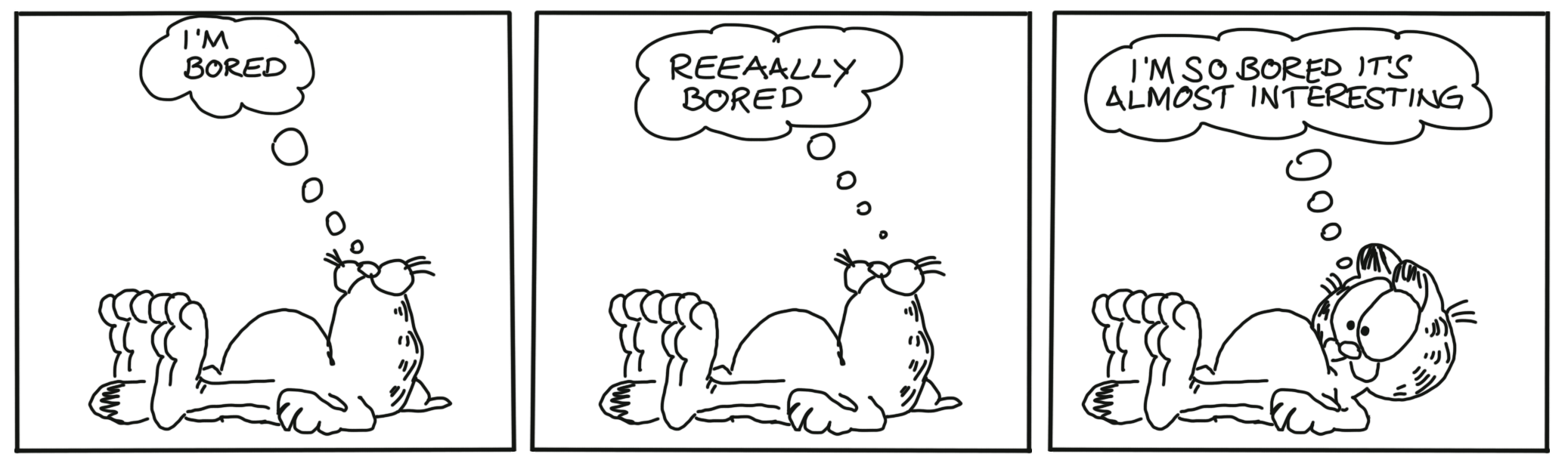Don't worry! Soon you won't be bored anymore!!

Totally content?

Then go grab a coffee, lean back and enjoy the rest of the day...!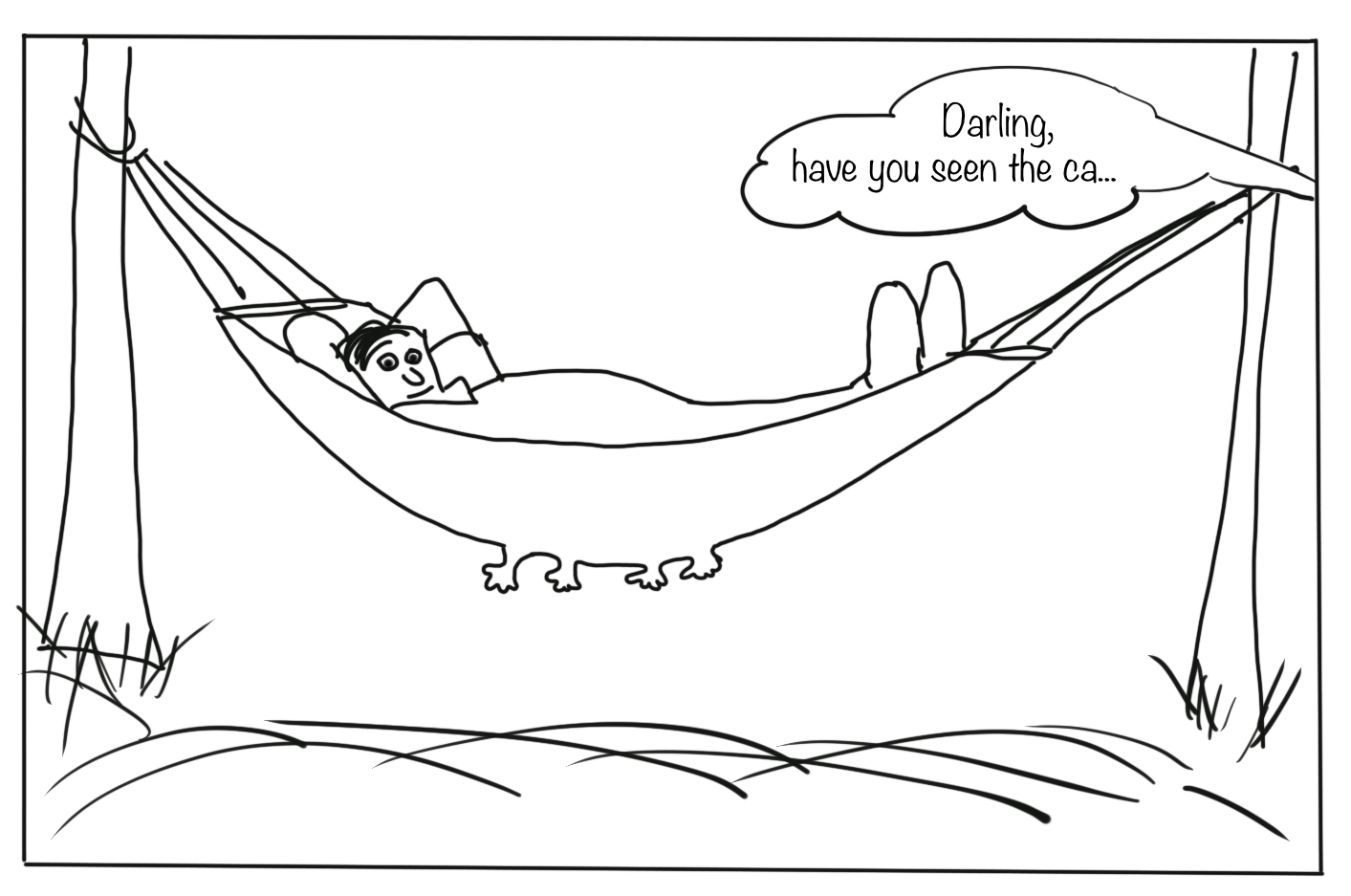Thank Youborrowed and mentioned with proper source: statements.
Image on title and end slide: Section of an infrared satallite image showing the Larsen C ice shelf on the Antarctic
Peninsula - USGS/NASA Landsat: A Crack of Light in the Polar Dark, Landsat 8 - TIRS, June 17, 2017
sel_group <- df$group == 2 # returns a logical vector df[sel_group, ] # column index is empty as we want all columns 2.Extract all observations where values are greater than 150. sel_value <- df$value > 150
sel_group <- df$group == 1 sel_value <- df$value < 50 | df$value > 150 df[sel_group & sel_value, ] 4.Extract all observations that have the letters "a", "c", "g", or "j". sel_sample <- df$sample %in% c("a", "c", "g", "j")